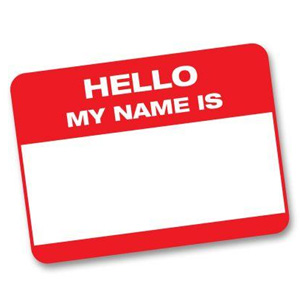# Practice Quiz On Nomenclature

33 Questions | Attempts: 4875
ShareSettingsNeeded SKILLS: Identifying types of chemical compounds, applying the rules to name or write the correct name or formula.

• 1.
Which of the following formulas represents a molecular compound?
• A.

SO2

• B.

ZnO

• C.

BeF2

• D.

Xe

• 2.
Which of the following shows both the correct formula and correct name of an acid?
• A.

HClO2, chloric acid

• B.

HNO2, hydronitrous acid

• C.

H3PO4, phosphoric acid

• D.

HI, iodic acid

• 3.
Which of the following shows a prefix used in naming binary molecular compounds with its corresponding number?
• A.

Hexa- , 8

• B.

Deca- , 7

• C.

Octa- , 4

• D.

Nona- , 9

• 4.
Suppose you encounter a chemical formula with H as the cation.  What do you know about this compound immediately?
• A.

It is probably an acid

• B.

It has a +1 charge

• C.

It is an ionic compound

• D.

It is a base

• 5.
Which of the following is not a cation?
• A.

Ca 2+

• B.

Sulfate

• C.

Iron (III) ion

• D.

Mercury (I) ion

• 6.
Which set of the chemical name and chemical formula for the compound is correct?
• A.

Iron (III) phosphate, FePO4

• B.

Ammonium sulfite, (NH4)2S

• C.

Lithium carbonate, LiCO3

• D.

Magnesium dichromate, MgCrO4

• 7.
What is the correct name for CoCl2
• A.

Cobalt (II) chlorate

• B.

Cobalt (I) chloride

• C.

Cobalt (I) chlorate

• D.

Cobalt (II) chloride

• 8.
When naming a transition metal ion that can have more than one common ionic charge, the numerical value of the charge is indicated by a
• A.

Superscript after the name

• B.

Roman Numeral following the anion

• C.

Prefix

• D.

Roman Numerals following the cation

• 9.
Which of the following correctly represents an ion pair and the ionic compound the ions would  form?
• A.

Na+, Cl- ; NaCl2

• B.

Ca +2, F- ; CaF2

• C.

Ba +2, O -2 ; Ba2O2

• D.

Pb +4 , O -2 ; Pb2O4

• 10.
What type of ions have names ending in -ide?
• A.

Only metal ions

• B.

Only cations

• C.

Only gaseous ions

• D.

Only anions

• 11.
Which element, when combined with fluorine, would most likely form an ionic compound?
• A.

Phosphorus

• B.

Lithium

• C.

Carbon

• D.

Chlorine

• 12.
Which of the following compounds contains the lead (II) ion?
• A.

Pb2O

• B.

PbO

• C.

Pb2S

• D.

PbCl4

• 13.
Which of the following is true about the composition of ionic compounds?
• A.

They are composed of anions and cations

• B.

They are formed from two or more nonmetallic elements

• C.

They are composed of anions only

• D.

They are composed of cations only

• 14.
What is the correct formula for barium chlorate?
• A.

BaCl2

• B.

Ba(ClO2)2

• C.

Ba(ClO3)2

• D.

Ba(ClO)2

• 15.
Which of the following shows correctly an ion pair and ionic compound the two ions form?
• A.

Fe +3 , O -2 ; Fe2O3

• B.

Cr +3 , I- ; CrI

• C.

Sn +4 , N -3 ; Sn4N3

• D.

Cu +2 , O -2 ; Cu2O2

• 16.
Which set of chemical name and formula for the same compound is correct?
• A.

Tin(IV)bromide ; SnBr4

• B.

Iron(II)oxide ; Fe2O3

• C.

Aluminum fluorate ; AlF3

• D.

Potassium chloride ; K2Cl2

• 17.
What is the correct name for Sn3(PO4)2 ?
• A.

Tin (V) phosphate

• B.

Tin (III) phosphate

• C.

Tritin diphosphate

• D.

Tin (II )phosphate

• 18.
Aluminum is a group 13 metal. Which ion does Al typically form?
• A.

Al -3

• B.

Al +5

• C.

Al +3

• D.

Al -5

• 19.
Which of the following formulas represents an ionic compound?
• A.

CS2

• B.

BaI2

• C.

PCl3

• D.

N2O4

• 20.
Molecular compounds are usually
• A.

Composed of two or more nonmetals

• B.

Composed of positive and negative ions

• C.

Composed of metal and nonmetal

• D.

Composed of two or more transition elements

• 21.
How are chemical formulas of binary ionic compounds generally written?
• A.

Anion on the left, cation on the right

• B.

Roman numeral first, then anion, then cation

• C.

Subscripts first, then ions

• D.

Cation on the left, anion on the right

• 22.
Which of the following correctly provides the names and formulas of polyatomic ions?
• A.

Nitrite: NO - ; nitrate: NO -2

• B.

Carbonate: HCO -3 ; bicarbonate: CO3 -2

• C.

Sulfite: S -2 ; sulfate: SO3 -

• D.

Chromate: CrO4 -2 ; dichromate: Cr2O7 -2

• 23.
What is the correct name for the chemical symbol N -3?
• 24.
What is the formula for sulfurous acid?
• 25.
What is the formula for hydrosulfuric acid?

## Related TopicsBack to top
×

Wait!
Here's an interesting quiz for you.